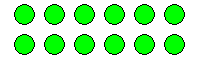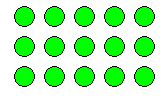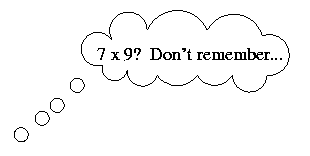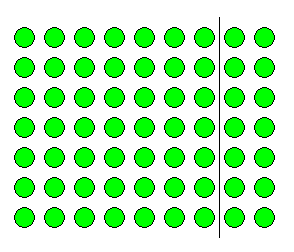# Multiplication Series: Illustrating Number Properties with Arrays

##### Age 5 to 11

Published 2002 Revised 2011

An introduction on using arrays can be found here.

Whether taught formally in school or not, the properties that apply to numbers in operations are encountered by children during their learning of mathematics. A sound understanding of these properties provides a good basis for developing operations, including mental calculation. The operation of addition comes easily to most children, but working with multiplication requires more sophisticated thinking and therefore usually needs more support. Modelling number properties involving multiplication using an array of objects not only allows children to represent their thinking with concrete materials, but it can also assist the children to form useful mental pictures to support memory and reasoning.### Commutative property

The commutative property of multiplication can be neatly illustrated using an array. For example, the array above could be read as $2$ rows of $6$, or as $6$ columns of $2$. Or the array could be physically turned around to show that $2$ rows of $6$ has the same number as $6$ rows of $2$. Regardless of the way you look at it, there remain $12$ objects. Therefore, the array illustrates that $2 \times6 = 6 \times 2$, which is an example of the commutative property for multiplication. Being able to apply the commutative property means that the number of multiplication facts that have to be memorised is halved.

### Division as the Inverse Operation of Multiplication

Of the four operations, division is the most troublesome for young students. Full understanding of division tends to lag well behind the other operations. For many children opportunities to explore the concept with concrete materials are curtailed well before they perceive the relationships between division and the other four operations. One such relationship, the inverse relationship between division and multiplication, can be effectively illustrated using arrays.For example; $3 \times5 = 15$ ($3$ rows of $5$ make $15$), can be represented by the following array. Looking at the array differently reveals the inverse, that is; $15 \div 3 = 5$ ($15$ put into $3$ rows makes $5$ columns - or $5$ in each row). Language clearly plays an important role in being able to express the mathematical relationships and the physical array supports this aspect of understanding by giving the students something concrete to talk about.

Placing the mathematics into a real-life context through word problems can facilitate both understanding of the relationship and its expression through words. For example, "The gardener planted $3$ rows of $5$ seeds. How many seeds did she plant?" poses quite a different problem to "The gardener planted $15$ seeds in $3$ equal rows. How many seeds in each row?" yet both these word problems can be modelled using the same array.

Further exploration of the array reveals two more ways of expressing inverse relationships: $5 \times3 = 15$ and $15 \div 3 = 5$ . The word problems can be adapted to describe these operations and highlight the similarities and differences between the four expressions modelled by the one array.

### Distributive property of multiplication over addition

This rather long title not only names one of the basic properties that govern our number system, it also names a personally invented mental strategy that many people regularly use. This strategy often comes into play when we try to recall one of the handful of multiplication facts that, for various reasons, are difficult to remember. For example, does this kind of thinking seem familiar?"I know $7 \times7$ is $49$.
I need two more lots of $7$, which is $14$.
So if I add $49$ and $14$... that makes $63$.
Ah yes! $7 \times9=63$.'"

Symbolically, this process can be represented as...

\begin{eqnarray} 7 \times 9 &=& 7 \times (7 + 2)\\ &=& (7 \times 7)+(7 \times 2) \\ &=& 49 + 14 \\ &=& 63 \end{eqnarray}

Another way to explain this process is through an array.1. The whole array represents $7 \times9$ ($7$ rows of $9$)
2. The smaller array to the left of the line shows $7 \times7$ ($7$ rows of $7$).
3. The small array to the right of the line shows $7 \times2$ ($7$ rows of $2$).
4. It can now be easily seen that $7 \times9$ is the same as $(7 \times 7) + (7 \times2)$, which leads to $49 + 14 = 63$.
A slightly different approach to looking at this partitioned array fully illustrates the distributive property by highlighting the first step of splitting the $9$ into $7 + 2$, before the multiplying begins. With the partition line in place, each individual row of the whole array represents $9 = 7 + 2$. Therefore, all $7$ rows represent $7 \times(7 + 2)$, and as can be seen on the array, this is the same as $(7 \times7) + (7 \times2)$.